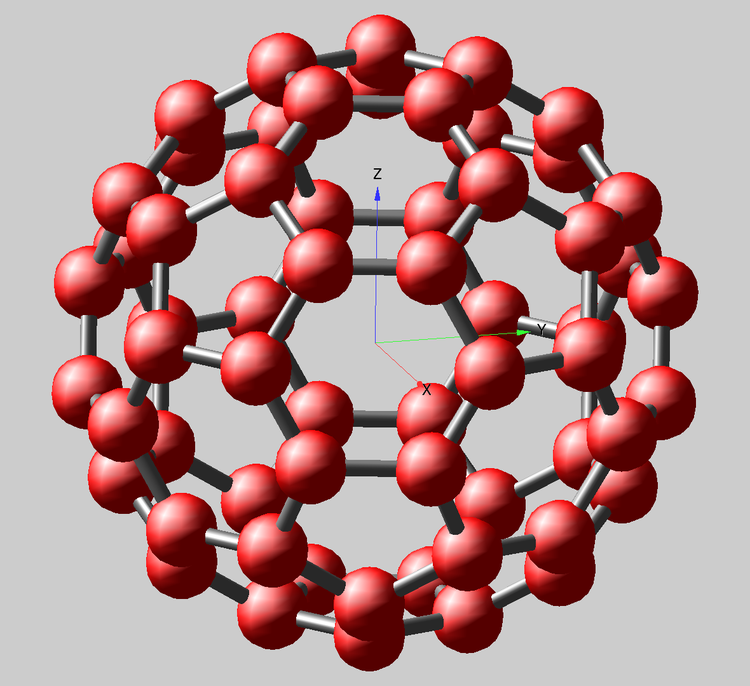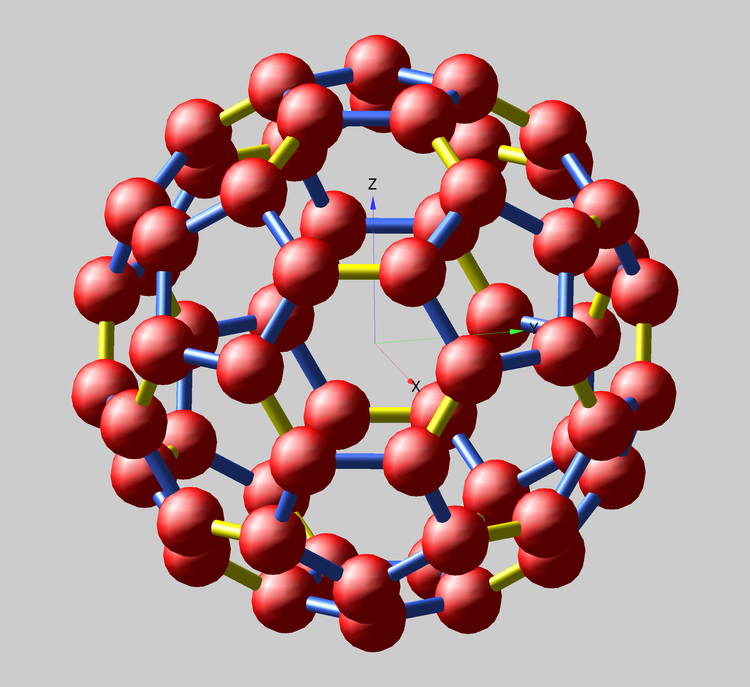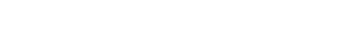# Molecular Bond Visualization with Gizmo in Igor Pro 7Submitted by Stephen Chinn, Ph.D. (EE)

This blog post is meant to show how Igor Pro 7 can be a useful tool for 3D visualization of some molecular bond structures having too many bonds to be input by manual entry. An earlier WaveMetrics example, Molecule.pxp, made with Igor Pro 6, had few molecules, and could not use Igor Pro 7's new Gizmo Path tube-visualization capability. Another useful topic described below is the use of Path waves which contain data for disparate bond segments, separated by NaN rows. I wish to thank AG (WaveMetrics) for suggesting the NaN path-gap idea, and visually enhancing the final Gizmo display.

The post is not meant to be a Gizmo tutorial; some basic familiarity with Gizmo usage is assumed. WaveMetrics provides extensive Help files and Examples on all of Gizmo's aspects if you need to come up to speed.

The simplest example that interested me is the fullerene molecule C60 (shown above; also called buckminsterfullerene, for the famous architect who came up with the "bucky-ball" structure). It is simple because there is only one type of atomic constituent, carbon. The structure is highly symmetric and has only two types of C-C bonds and bond lengths, which are either on pentagonal rings, or shared hexagon edges. This molecule is receiving new attention for its potential usage in very small atomic clocks:
https://spectrum.ieee.org/semiconductors/materials/to-build-the-worlds-smallest-atomic-clock-trap-a-nitrogen-atom-in-a-carbon-cage

A necessary starting point for the bonds' description is a knowledge of their x, y, and z coordinates.
If you want to exercise the code below and need the Cartesian coordinates for the C60 structure you can use the following link:
http://www.ccl.net/chemistry/resources/messages/2000/12/07.005-dir/
Look for the section section headed by:
Center     Atomic     Atomic              Coordinates (Angstroms)
Number     Number      Type              X           Y           Z

Copy and paste the 60 numerical columns below the section header into a plain-text file, which will have 60 row entries. Use the Igor Pro 7 Data→Load Waves→Load General Text... menu item. Select your text file, skip the first three columns, and load the last three columns into waves named 'wx', 'wy', and 'wz'. It will also be necessary to have a triplet N=(60,3) wave to construct the Gizmo Path object. this is easily accomplished by using a WaveMetrics utility procedure in your Procedure file:

`#include <XYZtoTripletToXYZ>`

The included ipf adds a Macro in the main Menu "XYZ waves to XYZ Triplet". Combine the three waves 'wx', 'wy', and 'wz' into a new triplet wave 'wxyz'. Next add to your Procedure window a user utility function that calculates the Cartesian distance between any two C atoms.

function dist(m,n)
variable m, n

WAVE wx, wy, wz
if(m==n)
return 0
else
return sqrt((wx[m]-wx[n])^2 + (wy[m]-wy[n])^2 + (wz[m]-wz[n])^2)
endif
end

Now we have to figure out which pairs of C atoms are nearest neighbors. There are two possible bond lengths, 1.45331 or 1.36705 Angstrom, for the data file given above. (If you use a different data set, you should recalculate possibly different bond lengths.) A useful summary of the nearest-neighbor locations is an Adjacency Matrix. In the present example the full matrix has N=(60,60), with values either 1 or 0, depending on whether the atoms at the matrix[p][q] indices are nearest neighbors or not.

Only the upper-right matrix corner is required, but for coding simplicity the full Adjacency Matrix is constructed in the following function. The test for being a nearest-neighbor pair is that the bond distance be >0 and less than the maximum bond length (plus a small tolerance factor). The final returned value is a check on the correct number of bonds (or 3D polygon edges).

WAVE wx, wy, wz
Adj = (dist(p,q)>0 && dist(p,q)<1.4534) ? 1 : 0
return msum/2         //  check the number of edges 3N/2
end

Finally, the Path object for visualizing the nearest-neighbor bonds is calculated. The Pathwave, 'wpath', is a series of two triplets connecting nearest-neighbors, with each pair separated by an additional NaN triplet. This expains the wave dimensions    N = (3*(Number of bonds), 3 coordinates/atom). In this function, only the upper-right corner of the Adjacency Matrix is used.

function fpath()    //  create a path wave for NaN-separated bond paths

WAVE wxyz   //  triplet coordinate wave

make/O/N=((90*3), 3) wpath  //  2 rows + 1 Nan per bond
variable m, n, ktr=0
for(m=0;m<60;m+=1)
for(n=m+1; n<60; n+=1)
wpath[ktr][]          = wxyz[m][q]
wpath[ktr+1][]  = wxyz[n][q]
wpath[ktr+2][]  =   NaN
ktr+=3
endif
endfor
endfor
end

Then the Gizmo display is created, based on the fundamental Scatter0 object using the triplet coordinate wave 'wxyz' and the Path0 object using the wave 'wpath'. The path uses Igor Pro 7's new Tube display mode. All other Gizmo additions are to enhance the display appearance. Although this example is simple in its symmetry and composition, it may suggest ways to apply similar Igor Pro 7 methods to visualizing more complicated molecules. For example, straightforward modification of the above functions enables creation of two separate adjacency matrices and paths for long (blue) and short (yellow) bonds. There are 60 long and 30 short bonds. Note that pentagonal rings contain only long bonds, while short bonds occur only at edges between shared hexagons.The details for the display shown at the top of the post are given in the following (simpler) recreation macro.

Window Gizmo0() : GizmoPlot
PauseUpdate; Silent 1       // building window...
// Building Gizmo 7 window...
NewGizmo/W=(62.25,49.25,652.5,590)
ModifyGizmo startRecMacro=700
ModifyGizmo scalingMode=8
ModifyGizmo setOuterBox={-4.5,4.5,-4.5,4.5,-4.5,4.5}
ModifyGizmo scalingOption=0
ModifyGizmo keepPlotSquare=1
AppendToGizmo Scatter=root:wxyz,name=scatter0
ModifyGizmo ModifyObject=scatter0,objectType=scatter,property={ scatterColorType,0}
ModifyGizmo ModifyObject=scatter0,objectType=scatter,property={ markerType,0}
ModifyGizmo ModifyObject=scatter0,objectType=scatter,property={ sizeType,0}
ModifyGizmo ModifyObject=scatter0,objectType=scatter,property={ rotationType,0}
ModifyGizmo ModifyObject=scatter0,objectType=scatter,property={ Shape,2}
ModifyGizmo ModifyObject=scatter0,objectType=scatter,property={ size,1}
ModifyGizmo ModifyObject=scatter0,objectType=scatter,property={ color,1,0,0,1}
ModifyGizmo modifyObject=scatter0,objectType=scatter,property={calcNormals,1}
AppendToGizmo freeAxesCue={0,0,0,0.4},name=freeAxesCue0
ModifyGizmo modifyObject=freeAxesCue0,objectType=freeAxesCue,property={calcNormals,1}
AppendToGizmo Path=root:wpath,name=path0
ModifyGizmo ModifyObject=path0,objectType=path,property={ pathColorType,1}
ModifyGizmo ModifyObject=path0,objectType=path,property={ lineWidthType,0}
ModifyGizmo ModifyObject=path0,objectType=path,property={ pathColor,0.466667,0.466667,0.466667,1}
ModifyGizmo ModifyObject=path0,objectType=path,property={ drawTube,1}
ModifyGizmo ModifyObject=path0,objectType=path,property={ calcNormals,1}
ModifyGizmo modifyObject=path0,objectType=Path,property={calcNormals,1}
ModifyGizmo setObjectAttribute={path0,blendFunc0}
ModifyGizmo setObjectAttribute={path0,specular0}
AppendToGizmo light=Directional,name=light0
ModifyGizmo modifyObject=light0,objectType=light,property={ position,-0.241800,-0.664500,0.707100,0.000000}
ModifyGizmo modifyObject=light0,objectType=light,property={ direction,-0.241800,-0.664500,0.707100}
ModifyGizmo modifyObject=light0,objectType=light,property={ ambient,0.133000,0.133000,0.133000,1.000000}
ModifyGizmo modifyObject=light0,objectType=light,property={ specular,1.000000,1.000000,1.000000,1.000000}
AppendToGizmo attribute blendFunc={770,771},name=blendFunc0
AppendToGizmo attribute specular={1,1,1,1,1032},name=specular0
AppendToGizmo attribute specular={1,1,0,1,1032},name=specular1
AppendToGizmo attribute shininess={5,20},name=shininess0
AppendToGizmo attribute specular={1,1,0,1,1032},name=specular2
AppendToGizmo attribute shininess={5,20},name=shininess1
ModifyGizmo setDisplayList=0, object=freeAxesCue0
ModifyGizmo setDisplayList=1, object=light0
ModifyGizmo setDisplayList=2, attribute=shininess1
ModifyGizmo setDisplayList=3, attribute=specular2
ModifyGizmo setDisplayList=4, object=path0
ModifyGizmo setDisplayList=5, object=scatter0
ModifyGizmo setDisplayList=6, opName=clearColor, operation=clearColor, data={0.8,0.8,0.8,1}
ModifyGizmo currentGroupObject=""
ModifyGizmo showInfo
ModifyGizmo infoWindow={911,4,1724,245}
ModifyGizmo endRecMacro
ModifyGizmo SETQUATERNION={-0.479222,-0.350733,-0.486510,-0.640841}
EndMacro

Stephen Chinn, Ph.D. (EE)ForumSupportGallery